# Calculate File

The calculate.js file is where all the action happens. The calculate file uses javascript. This is where you write the logic that performs the necessary transformations on your input to create your desired output. The calculate.js file must always return an output object based on the format specified below. The calculate.js file also handles all reporting functions, and returns the report that is generated by your calculator.

### Layout#

module.exports = function (input_json) {
// Get Input
let { a, b } = input_json;
// Perform Logic
// Per Output Format
let output = {};
// Return Output
return output;
}

### Output Format#

{
"report": REPORT,
"results": {
"Result A": {
"value": a,
"units": "m",
},
"Result B": {
"value": b,
"units": "kN",
},
},
"ur_a": {
"label":"Utility Ratio A", //you can add this label key if you want to use cleaner keys
"value": 1.282,
"units": "utility",
},
"ur_b": {
"label":"Utility Ratio B",
"value": 0.751,
"units": "utility", //this can then be read by the UI to show PASS/FAIL
}
}
}

## ReportHelpers#

### ReportHelpers.round()#

Will round any number to 2 decimal places, or if you provide a second argument it will round to that figure. It will work with strings and handle NaNs without crashing.

This will return a number back, that way you can continue to use this as a number (not a string)

#### Examples#

ReportHelpers.round(0.432731); // 0.43
ReportHelpers.round(0.432731, 4); // 0.4327
ReportHelpers.round("1.736242"); // 1.74

### ReportHelpers.convert()#

Converts units

#### Examples#

ReportHelpers.convert(10, "in", "mm"); //254
ReportHelpers.convert(10, "kN", "kip"); //2.2480894244319
ReportHelpers.convert(2352, "mm", "m"); //2.352

### ReportHelpers.formula()#

Will automatically evaluate and print an equation to represent the result.

ReportHelpers.formula(options)

#### Example#

var moi = ReportHelpers.formula({
"formula": "I_y = b * Math.pow(h, 3) / 12",
"variables": { //must include the variable values
"b": b,
"h": h,
},
"units": "mm^4",
"dec_places": 2,
"intermediate_result": true, //will show an intermediate equation with the actual numbers
"reference": "Clause 1.2.3 AISC"
}); // will return the value of moi

#### Output#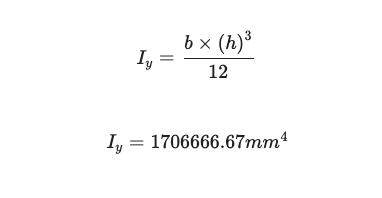### ReportHelpers.image()#

Will print an image in the report

ReportHelpers.image(options)

#### Example#

ReportHelpers.image({
new_block: true, //if you already have a block open, set this to false
})

### ReportHelpers.lineResult()#

This will create a nice easy and lightweight reporting option. Rather than showing full equations, you might just want to print intermediate results:

ReportHelpers.formula(description, symbol, value)

Note: You will need to open a REPORT block for this to be visible:

#### Examples#

REPORT.block.new()
ReportHelpers.lineResult("Max Shear force in Member", "V_{y}", 22.43 + " kN")
ReportHelpers.lineResult("Displacement Max", "\\gamma_y", max_def + " mm")
ReportHelpers.lineResult("Design Load", "\\omega_x", 77.43 + " kNm")
ReportHelpers.lineResult("Section Capacity", "\\gamma_{y2}", 123.43 + " kNm")
ReportHelpers.lineResult("Remaining Capacity in Section", "\\gamma_{y3}", 46 + " kNm")
ReportHelpers.lineResult("Design Load", "\\omega_x", 77.43 + " kNm")
ReportHelpers.lineResult("Section Capacity", "\\gamma_{y2}", 123.43 + " kNm")
ReportHelpers.lineResult("Remaining Capacity in Section", "\\gamma_{y3}", 46 + " kNm")
REPORT.block.finish()

#### Output#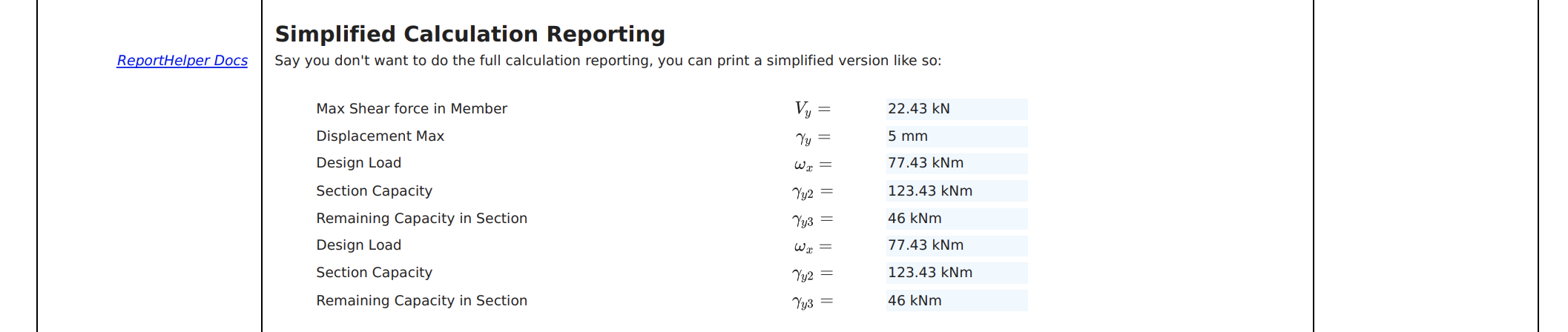### ReportHelpers.quickTable()#

#### Example#

// QUICK TABLES
let table_data = [
["Some Result", "1223", "kN"],
["Some Other Result", "0.234", "MPa"],
["Some Result", "1223", "kN"],
["Some Other Result", "0.234", "MPa"],
["Some Other Result", "0.234", "MPa"],
]
let options = {
widths: ["50%", "25%", "25%"],
text_aligns: ["left", "right", "center"], //or just "center"
reference: "Clause 1.2.3 AISC"
}
ReportHelpers.quickTable(table_data, options);

#### Output#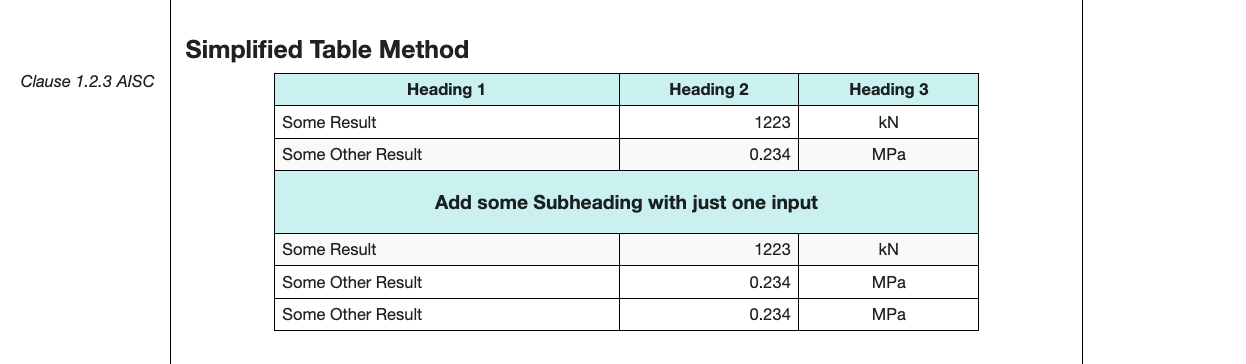### ReportHelpers.printStatus()#

This will automatically format a clean colour-based result on the right side of the table. It will automaticall read a value, and if it is > 1 it will show a red FAIL. If it is < 1, it will show a green PASS:

REPORT.block.new();
ReportHelpers.printStatus(0.128, true);
REPORT.block.finish();
//OR - if you want to create a new block
ReportHelpers.printStatus(0.888, false);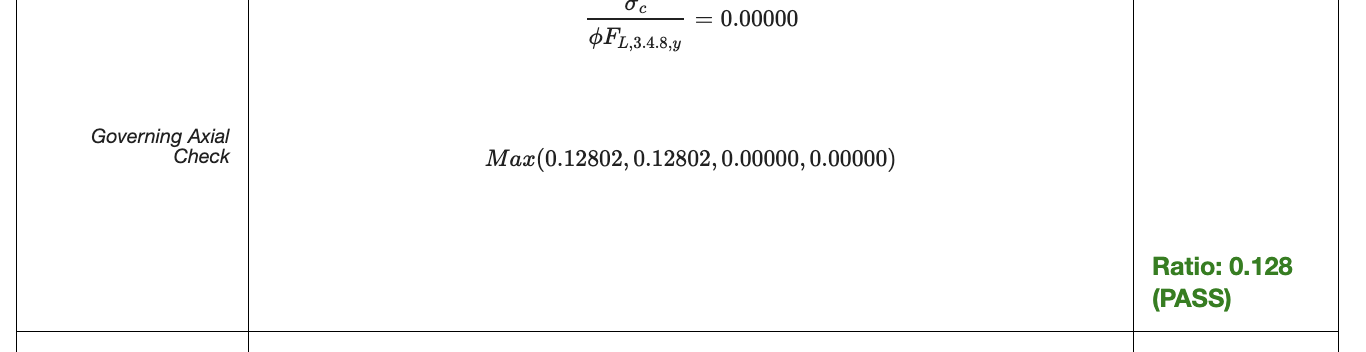## Other Packages#

The following packages are also installed on the report by default:

• VariableCollection = calculate.js supports any variable functionality
• ResultsTable - create results tables
• SectionSVG = SVGs can be drawn using SkyCiv Section SVG Package (see dev-docs)
• Graph = (see dev-docs)
• JsToLatex = (see dev-docs)
• calculate = (see dev-docs)

## How to Debug your Code#

Since the code is running on our server (under a controlled environment) it may be difficult to debug. Errors are caught and returned to the user as a notification and in the actual report: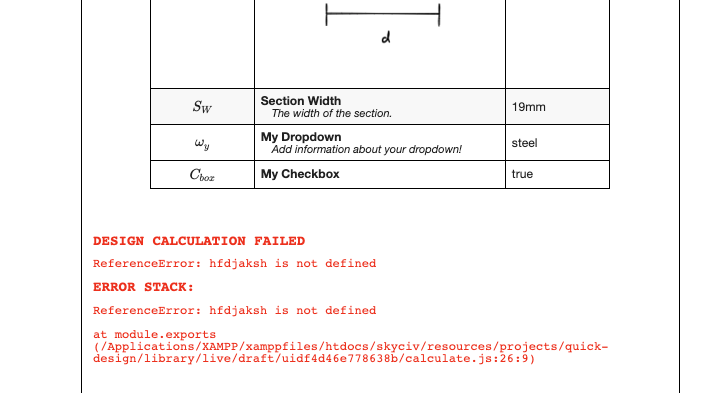### Logging:#

logger("E = " + youngs_modulus); // log some values or results
logger("ENTERED IF STATEMENT"); // helpful for debugging and quick logging
logVariables({shape, welded,alloy,Mz}) //will print a batch of variables like so:
shape = hollow rectangular
welded = Yes
alloy = 6005
Mz = 0

When you run your calculations, these will be available in the top corner of your code: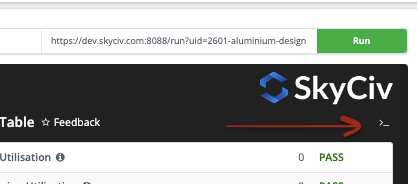Having a detailed log will help both you and the user identify issues in the code: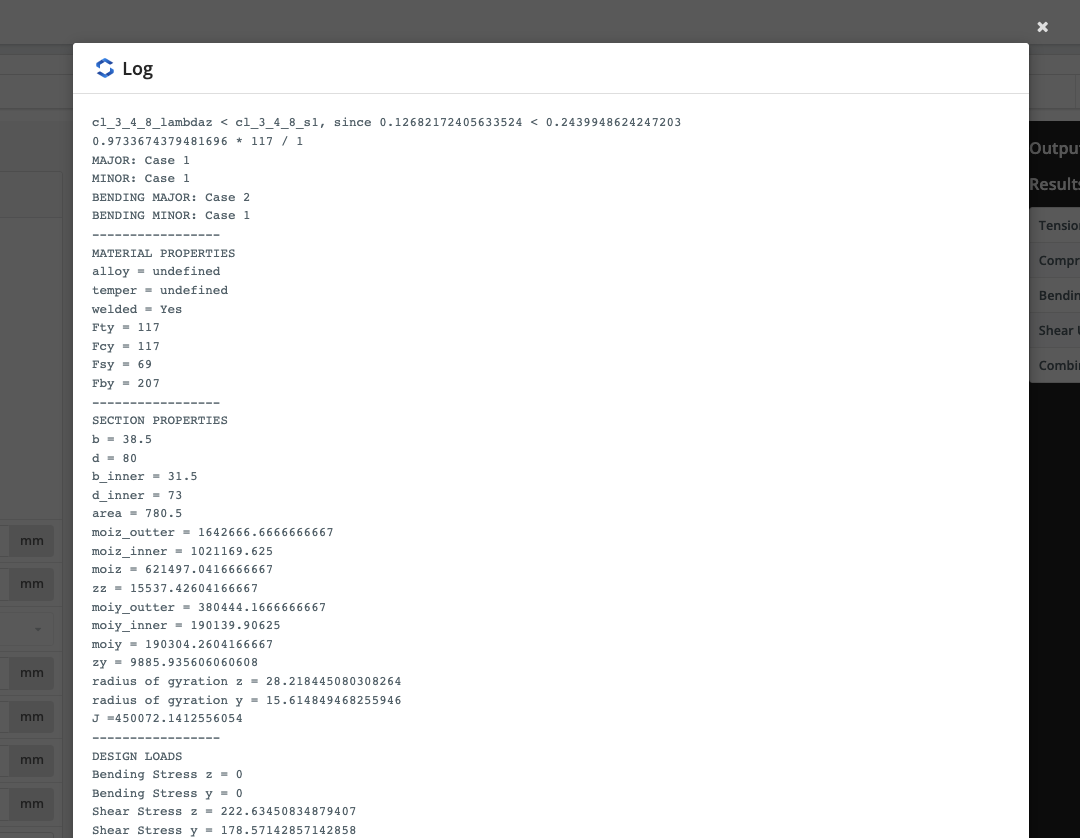## FULL EXAMPLE#

module.exports = function (input_json) {
ReportHelpers.printInputSummary();
ReportHelpers.print("By Pythagoras Theorem");
let a_squared = Math.pow(a, 2);
let b_squared = Math.pow(b, 2);
let c = Math.sqrt(( a_squared + b_squared )).toFixed(2);
REPORT.block.new();
REPORT.block.addCalculation('[math] C^2 = A^2 + B^2 \\therefore C = \\sqrt{ A^2 + B^2 } [math]');
REPORT.block.addCalculation('[math] C = \\sqrt{ ' + a + '^2 + ' + b + '^2 } [math]');
REPORT.block.addCalculation('[math] C = \\sqrt{ ' + (a_squared + b_squared) + ' } = ' + (c) + '[math]');
REPORT.block.finish();
var output = {
"pass": true,
"report": REPORT,
"results": {
"Missing Side (C)": {
"value": c,
"units": "m",
},
}
}
return output;
}